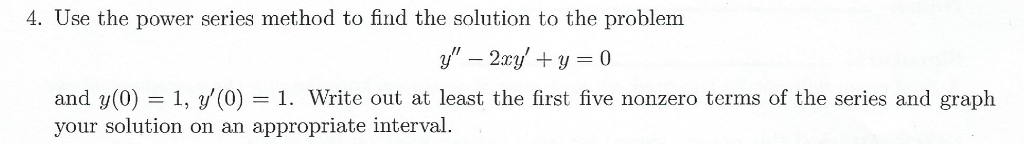Solved: 4. Use The Power Series Method To Find The Solution To The Problem Y- 2xyy 0 And Y(0) 1, Y'(0) = 1. Write Out At Least The First Five Nonzero Terms Of The Series And Graph Your Solution On An

By |4. Use the power series method to find the solution to the problem y- 2xyy 0 and y(0) 1, y'(0) = 1. Write out at least the first five nonzero terms of the series and graph your solution on an appropriate interval